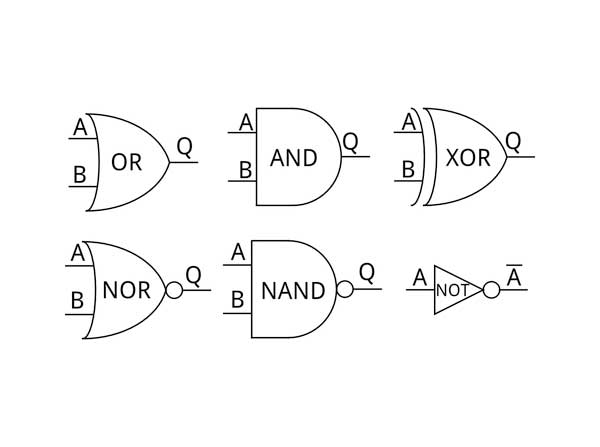﻿ Introduction to Logic Gates | EngineersGarage
Close or Esc Key

Introduction to Logic Gates

You must have heard that calculators and computers store decimal numbers in the form of binary code as long strings of Zeroes and Ones.  In internal circuitry, each digit (0 or 1) is stored in electronic switches called transistors. It is easy to store binary numbers simply by switching the transistor On and Off. We can say that switching ON the transistor represents number 1 and switching OFF represents number 0.  But how can we add, multiply, subtract or perform various mathematical operations using just electrical currents? This can be done by using smart and logical circuits known as Logic Gates.

What are Logic Gates?

The word “logic” itself gives us an idea that they work on certain kind of logical operations. A gate can keep us restricted or can allow us to go out. In a similar manner, logic gate can either restrict electricity or let it flow.  As from childhood, we have learnt about logical operations in mathematics such as +, *, –, etc. Similarly, we can relate mathematical logical operations with the operations of logic gates.

Logic gates are the basic building blocks of digital electronics which have 1 or more input signals based on which it gives one logical output. Logic gate works on digital signals only and there are two states of these signals i.e. 0 as low and 1 as high or ON and OFF state. That’s why Logic Gates are known as digital electronic devices. The logical state of any terminal of gate can change its state, and it does generally change, as processing is happening in the circuit.  A table which gives the relation between different input signals corresponding to its output is known as Truth Table. By the help of this truth table we can identify how logic circuit works in response with different input signals which we apply to logic gates. An example of truth table of AND gate can be seen below where output is “1” only when both the inputs are “1” else the output is 0.

INPUTINPUTOUTPUT
ABY
000
010
100
111

There are two types of logic state, namely positive logic and negative logic. Positive logic is generally used by most of the electronics devices, whereby we consider higher voltage levels as 1 and lower voltage levels as 0. For example, in logic gates we consider +5V as 1 and from range 0V to 0.49V as 0. Whereas in negative logic we take high voltage levels as 0 and low voltage levels as 1.

Based on the input-output relation we have different kinds of logic gates. Three basic logic gates are as follows OR gate, AND gate and NOT gate. Others are NAND, NOR, XOR and ExOR gates.Fig. 1: Symbols of Logic Gates

By using different combinations of Logic gates, many advanced and complex circuits can be achieved. Theoretically, there is no limit on the number of gates to be arrayed in a single circuit but practically, only limited number of gates can be arranged in a given physical space. Different ICs contain arrangements of different logic gates. Also, as logical gates are mathematical symbols, it is possible to do any sort of calculation by the arrangement of different logic gates.